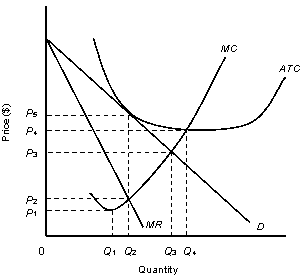/
/
/
41.Suppose you inherit the only spring of mineral water in
Not my Question
Flag Content

# Question : 41.Suppose you inherit the only spring of mineral water in : 1413822

41.Suppose you inherit the only spring of mineral water in an area and want to maximize profits from this costless product. You would ask your customers to bring their containers with them and:

a.charge them the highest price possible to sell some output.

b.charge them the lowest price possible to sell as much as you can.

c.ask them how much they would like to pay and accept it.

d.charge the price at which MR is zero.

e.charge the price at which MR is maximum.

42.If at the profit-maximizing level of output, a monopolist’s average-total-cost curve lies above its demand curve, then:

a.the firm should shut down in the short run.

b.the firm is earning economic losses.

c.the firm is earning economic profits.

d.the firm should increase its output.

e.the firm should decrease its output.

NARRBEGIN: Figure 10.3

The following figure shows the revenue and cost curves of a monopolist.

Figure 10.3D: Average Revenue

MR: Marginal Revenue

ATC: Average Total Cost

MC: marginal Cost

NARREND

43.Consider the monopolist described in Figure 10.3. If the firm engages in profit-maximizing behavior, what price will it charge?

a.P1

b.P2

c.P3

d.P4

e.P5

44.Consider the monopolist described in Figure 10.3. The firm can maximize profit by producing:

a.zero units.

b.Q1 units.

c.Q2 units.

d.Q3 units.

e.Q4 units.

45.Consider the monopolist described in the Figure 10.3. If the firm engages in profit-maximizing behavior, economic profit per unit of output will be:

a.0

b.P2

c.P4 minus P2

d.P5 minus P4

e.P5 minus P1

NARRBEGIN: Table 10.4

The table given below shows the price, marginal revenue and marginal cost of a monopolist at different levels of the output. The firm does not incur a fixed cost of production.

Table 10.4

Quantity SoldPrice per UnitMarginal RevenueMarginal Cost

0\$17\$0\$0

1\$16\$16\$4

2\$15\$14\$6

3\$14\$12\$8

4\$13\$10\$10

5\$12\$8\$12

NARREND

46.Refer to Table 10.4. Assuming that the monopolist is maximizing profits, the price the monopolist will charge is _____.

a.\$13.

b.\$14.

c.\$12.

d.\$16.

e.\$15.

47.Refer to Table 10.4 and calculate the total revenue earned by the monopolist at the profit maximizing level of output.

a.\$60.

b.\$16.

c.\$52.

d.\$42.

e.\$30.

48.In Table 10.4, assume that total fixed costs are \$20. What is the maximum profit the firm can earn at equilibrium?

a.\$0

b.\$4

c.\$16

d.\$30

e.\$52

49.If the monopolist’s price happens to be greater than the average-variable cost but less than the average total cost, in the short run the monopolist will:

a.be forced to shut down to minimize the cost.

b.operate at a loss.

c.operate at an economic profit.

d.operate at a normal profit.

e.go out of business.

50.Why is there a supply point and not a supply curve for a monopolist?

a.A monopolist cannot affect the market price by changing its supply.

b.A monopolist produces a homogeneous product having similar substitutes

c.A monopolist equates the price which it charges with its marginal cost

d.There is only one quantity and price at which a monopolist operates

e.A monopolist supplies to a large number of consumers

## Solution 5 (1 Ratings )

Solved
Economics 1 Year Ago 26 Views
This Question has Been Answered!